# KSEEB Solutions for Class 8 Maths Chapter 5 Squares, Square Roots, Cubes, Cube Roots Ex 5.7

In this chapter, we provide KSEEB SSLC Class 8 Maths Chapter 5 Squares, Square Roots, Cubes, Cube Roots Ex 5.7 for English medium students, Which will very helpful for every student in their exams. Students can download the latest KSEEB SSLC Class 8 Maths Chapter 5 Squares, Square Roots, Cubes, Cube Roots Ex 5.7 pdf, free KSEEB SSLC Class 8 Maths Chapter 5 Squares, Square Roots, Cubes, Cube Roots Ex 5.7 pdf download. Now you will get step by step solution to each question.

## Karnataka Board Class 8 Maths Chapter 5 Squares, Square Roots, Cubes, Cube Roots Ex 5.7

Question 1.
Find the cube root by prime factorisation,
i) 1728
ii) 3375
iii) 10648
iv) 46656
v) 15625
i) 1728 = 2 × 2 × 2 × 6 × 6 × 6 = 12 × 12 × 12
1728−−−−√3=12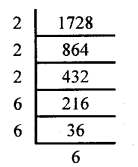ii) 3375 = 5 × 5 × 5 × 3 × 3 × 3 = 15 × 15 × 15
3375−−−−√3=15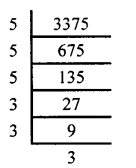iii) 10648 = 2 × 2 × 2 × 11 × 11 × 11
= 2 × 11 × 2 × 11 × 2 × 11
= 22 × 22 × 22
10648 = 223
∴ 10648−−−−−√3=22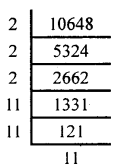iv) 46656 = 2 × 2 × 2 × 2 × 2 × 2 × 3 × 3 × 3 × 3 × 3 × 3
= 4 × 4 × 4 × 9 × 9 × 9
= 4 × 9 × 9 × 4 × 9 × 4
46656 = 36 × 36 × 36 = 363
∴ 46656−−−−−√3=36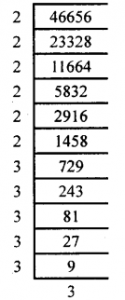v) 15625 = 5 × 5 × 5 × 5 × 5 × 5
= 25 × 25 × 25
15625 = 253
∴ 15625−−−−−√3=25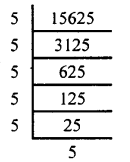2. Find the cube root of the following by looking at the last digit and using estimation.

Question (i)
91125
Unit digit of 91125 in 5. Therefore the units digit in its cube root is 5.
Let us split 91125 as 91 and 125. We find that 43 = 64 < 91 < 125 = 53
Hence 403 = 64000 <91125 < 125000 = 503
∴Cube root of 91125 lies between 40 and 50 and units digit is 5 the only such number is 45.
∴ 91125−−−−−√3=45

Question (ii)
166375
Units digit of 166375 is 5. Therefore the units digit of its cube root is 5.
Let us split 166375 as 166 and 375.
53 = 125 < 166 < 216 = 63
Hence 503 = 125000, < 166375 < 216000 = 603
∴ 166375−−−−−−√3 lies between 50 and 60. .
Since the units digit is 5 the only such number in 55.
∴ 166375−−−−−−√3=55

Question (iii)
704969
The units digit of 704969 is 9. Therefore the units digits of its cube root are 9.
Let us split 704969 as 704 and 969
83 = 512 < 704 < 729 – 93
Hence = 803= 512000 < 704969 < 72900 = 903
∴ 704969−−−−−−√3 lies between 80 and 90.
Since the units digits is 9 the only such number is 89.
∴ 704969−−−−−−√3=89

3. Find the nearest integer to the cube root of each of the following.
(i) 331776
(ii) 46656
(iii) 373248

Question (i)
331776
603 = 216000 < 331776 < 343000 = 703
Hence 331776−−−−−−√3 lies between 60 and 70.
We do not know whether 331776 in a perfect cube or not.
However we may sharpen the bound.
683 = 314432, 693 = 328509
Hence 331776−−−−−−√3 lies between 69 and 70
331776 – 328509 = 3267
343000 – 331776 = 11224
331776 in nearer to 693
∴ The closest integer to 331776−−−−−−√3 is 69.

Question (ii)
46656
303 = 2700 < 46656 < 64000 – 40<sub>3</sub>
46656−−−−−√3 lies between 30 and 40 we do not know whether 46656 in a perfect cube or not. However we may sharper the bound
353 = 42, 875, 363 = 46656
∴ 46656−−−−−√3=36

Question (iii)
373248
703 = 343000 < 373248 < 512000 – 803
373248−−−−−−√3 lies between 70 and 80. We do not know whether 373248 is a perfect cube or not.
However we may sharpen the bound. 713 = 357911, 723 = 373248
∴ 373248−−−−−−√3=72

All Chapter KSEEB Solutions For Class 8 maths

—————————————————————————–

All Subject KSEEB Solutions For Class 9

*************************************************

I think you got complete solutions for this chapter. If You have any queries regarding this chapter, please comment on the below section our subject teacher will answer you. We tried our best to give complete solutions so you got good marks in your exam.

If these solutions have helped you, you can also share kseebsolutionsfor.com to your friends.

Best of Luck!!